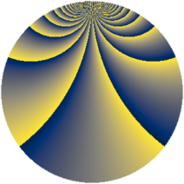# Properties

 Label 1148.2.cjLevel $1148$ Weight $2$ Character orbit 1148.cj Rep. character $\chi_{1148}(17,\cdot)$ Character field $\Q(\zeta_{120})$ Dimension $896$ Sturm bound $336$

# Learn more about

## Defining parameters

 Level: $$N$$ $$=$$ $$1148 = 2^{2} \cdot 7 \cdot 41$$ Weight: $$k$$ $$=$$ $$2$$ Character orbit: $$[\chi]$$ $$=$$ 1148.cj (of order $$120$$ and degree $$32$$) Character conductor: $$\operatorname{cond}(\chi)$$ $$=$$ $$287$$ Character field: $$\Q(\zeta_{120})$$ Sturm bound: $$336$$

## Dimensions

The following table gives the dimensions of various subspaces of $$M_{2}(1148, [\chi])$$.

Total New Old
Modular forms 5568 896 4672
Cusp forms 5184 896 4288
Eisenstein series 384 0 384

## Trace form

 $$896q + 8q^{9} + O(q^{10})$$ $$896q + 8q^{9} - 24q^{15} - 36q^{17} + 24q^{19} + 8q^{21} - 32q^{29} - 60q^{33} + 40q^{35} + 36q^{37} - 16q^{43} - 24q^{47} - 8q^{49} + 36q^{51} + 24q^{53} + 112q^{57} + 92q^{63} - 76q^{65} - 16q^{67} + 80q^{71} + 36q^{73} - 252q^{75} + 48q^{77} - 4q^{79} - 16q^{85} - 60q^{87} - 192q^{89} + 112q^{91} + 16q^{93} + 48q^{95} - 208q^{99} + O(q^{100})$$

## Decomposition of $$S_{2}^{\mathrm{new}}(1148, [\chi])$$ into newform subspaces

The newforms in this space have not yet been added to the LMFDB.

## Decomposition of $$S_{2}^{\mathrm{old}}(1148, [\chi])$$ into lower level spaces

$$S_{2}^{\mathrm{old}}(1148, [\chi]) \cong$$ $$S_{2}^{\mathrm{new}}(287, [\chi])$$$$^{\oplus 3}$$$$\oplus$$$$S_{2}^{\mathrm{new}}(574, [\chi])$$$$^{\oplus 2}$$